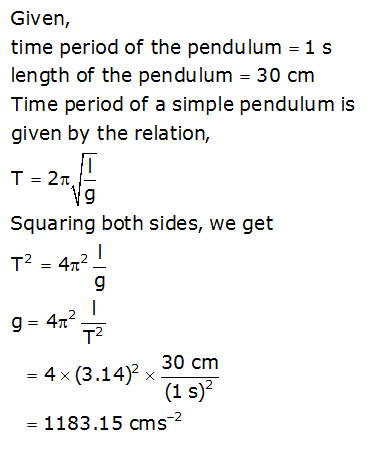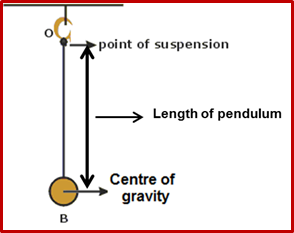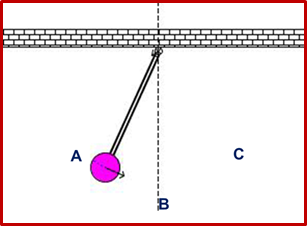# Measurement of Time

## •    Time is defined as an interval between two events. Second (s) is considered as the standard unit of time. Many clocks work on the principle of simple pendulum. •    Simple pendulum consists of a heavy, point mass suspended by an inextensible or non-elastic thread from a fixed point. The suspended heavy point mass is known as bob and it exhibits oscillatory motion. The time taken by the pendulum to make one complete oscillation is called time period and is measured in seconds.  The number of oscillation made by pendulum in one second is called the frequency of oscillation and is measured in hertz. Frequency is the reciprocal of the time period of the simple pendulum and vice-versa. •    If a graph is plotted between length and time, taking length on x-axis and time on y-axis, a non-straight line graph is obtained.•    If a graph is plotted between square root of the effective length and time period of the pendulum then a straight line inclined to x-axis is obtained.The value of acceleration due to gravity (g) can be calculated using the slope of the straight line graph. •    The time period of a simple pendulum is directly proportional to the square root of the effective length of the pendulum and is inversely proportional to the square root of the acceleration due to gravity. The time period of a simple pendulum is independent of the mass of the pendulum bob. •    Second’s pendulum is a simple pendulum with a time period of two seconds. The effective length of Second’s pendulum is one hundred centimetres.

To Access the full content, Please Purchase

• Q1

A simple pendulum is brought to a planet having acceleration due to gravity (g) different from the earth. If the time period of the pendulum is 1 s and its effective length is 30 cm, calculate the value of g on that planet.

Marks:2• Q2

Define time period and frequency of a simple pendulum.

Marks:2

Time Period: Time taken by the bob of a simple pendulum to complete one oscillation about its mean position is called time period pf the simple pendulum.

Frequency: It is defined as the number of oscillations completed by the pendulum per unit time.

• Q3

What is length of a simple pendulum?

Marks:1The distance between the centre of gravity (centre point) of the bob and the point of suspension of the bob is called length of the pendulum.

• Q4

Define amplitude of a simple pendulum.

Marks:1

Amplitude of a simple pendulum is defined as the maximum displacement through which a pendulum bob moves on either of its sides from its mean position.